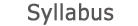2019 - 2020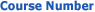0540-5001-01
Mathematical Methods in Engineering
FACULTY OF ENGINEERING | School of Mechanical Engineering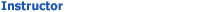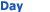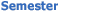Toledo Yaron Wolfson - Engineering 438 Wed 1600-1900 Sem  1
University credit hours:  3.0

Course description
Credit Points: 3
Vector and tensor algebra: definitions, representations of tensors, examples. Complex functions: definitions, singular points, the Cauchy theorem and applications, conformal maps and applications, expansions of integrals, the Cauchy integral, the Hilbert arc problem. Integral transforms: Fourier, Laplace, Mellin, Hankel and Hilbert, applications to ordinary differential equations (separation of variables) and partial differential equations. Fredholm and Volterra integral equations. Introduction to the calculus of variations.

accessibility declaration